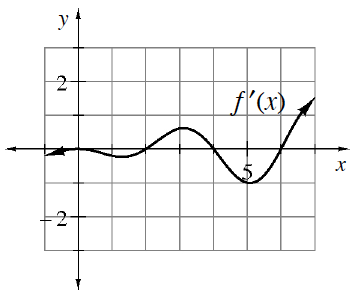### Home > APCALC > Chapter 11 > Lesson 11.4.1 > Problem11-110

11-110.

Multiple Choice: Based on the graph of $y = f ′\left(x\right)$ shown at right, $f$ must have a relative minimum at:

1. $x = 0$

1. $x = 2$

1. $x = 5$

1. $x = 6$

1. $x = 2$ and $6$The given graph is a slope graph. A minimum occurs where the slope graph changes from negative to positive.